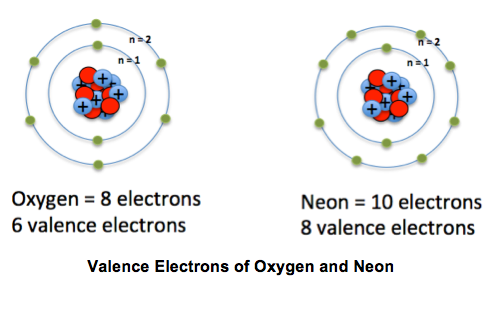What are some examples of valence electrons?

Apr 22, 2014

The valence electrons are the electrons that determine the most typical bonding patterns for an element.

These electrons are found in the s and p orbitals of the highest energy level (row of the periodic table) for the element.

Using the electron configuration for each element we can determine the valence electrons.

Na - Sodium $1 {s}^{2} 2 {s}^{2} 2 {p}^{6} 3 {s}^{1}$
Sodium has 1 valence electron from the 3s orbital

P - Phosphorus $1 {s}^{2} 2 {s}^{2} 2 {p}^{6} 3 {s}^{2} 3 {p}^{3}$
Phosphorus has 5 valence electrons 2 from the 3s and 3 from the 3p

Fe - Iron $1 {s}^{2} 2 {s}^{2} 2 {p}^{6} 3 {s}^{2} 3 {p}^{6} 4 {s}^{2} 3 {d}^{6}$
Iron has 2 valence electrons from the 4s

Br - Bromine $1 {s}^{2} 2 {s}^{2} 2 {p}^{6} 3 {s}^{2} 3 {p}^{6} 4 {s}^{2} 3 {d}^{10} 4 {p}^{5}$
Bromine has 7 valence electrons 2 from the 4s and 5 from the 4p
you can count the electrons in the outermost shell